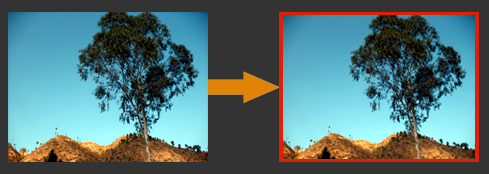Showing posts with label Image. Show all posts
Showing posts with label Image. Show all posts## Grayscale image

In our earlier tutorials we have seen about re-sizing image, drawing image border. On same way we will see about how to convert RGB image to Grayscale image using java code. When we talk about RGB to Grayscale we can convert in lot of ways like pixel by pixel, using Java API etc., Even in our below same codes we have tried with 2 ways like using Java Graphics class and next manually reading pixel by pixel and changing the RGB values.
First lets see by using Graphics class

```import java.awt.Graphics;
import java.awt.image.BufferedImage;
import java.io.File;
import java.io.IOException;
import javax.imageio.ImageIO;

public class ColorToGray {

public static void main(String[] args) {

try {
File colorImage = new File("D://color.png");
File grayImage = new File("D://gray.jpg");

BufferedImage image = new BufferedImage(cImage.getWidth(), cImage.getHeight(), BufferedImage.TYPE_BYTE_GRAY);
Graphics graphics = image.getGraphics();
graphics.drawImage(cImage, 0, 0, null);
graphics.dispose();

ImageIO.write(image, "jpg", grayImage);

} catch (IOException e) {
e.printStackTrace();
}
}
}
```

OUTPUT:

INPUT FILE                                                             OUTPUT FILE

Next we will see about reading pixel by pixel and converting RGB to Grayscale which will be time consuming but where we can play around with the pixels value which we want.

```import java.awt.Color;
import java.awt.image.BufferedImage;
import java.io.File;
import java.io.IOException;
import javax.imageio.ImageIO;

public class Grayscale {

public static void main(String[] args11) throws IOException {

File colorImage = new File("D://color.jpg");
String grayImage = "D://gray.jpg";

BufferedImage gImage = new BufferedImage(cImage.getWidth(), cImage.getHeight(), cImage.getType());

for(int i=0; i<cImage.getWidth(); i++) {

for(int j=0; j<cImage.getHeight(); j++) {

int alpha = new Color(cImage.getRGB(i, j)).getAlpha();
int red = new Color(cImage.getRGB(i, j)).getRed();
int green = new Color(cImage.getRGB(i, j)).getGreen();
int blue = new Color(cImage.getRGB(i, j)).getBlue();

int gray = (red + green + blue) / 3;
red = green = blue = gray;
gray = (alpha << 24) + (red << 16) + (green << 8) + blue;

gImage.setRGB(i,j,gray);

}
}

ImageIO.write(gImage, "jpg", new File(grayImage));
}
}
```

OUTPUT:

INPUT FILE                                                             OUTPUT FILE## Drawing image border using Java Code

In our last tutorial we have seen how to re-size image using Java code. In this tutorial we will see how to draw a border in the image using Java code.

import java.awt.image.BufferedImage;
import java.io.File;
import java.io.IOException;
import javax.imageio.ImageIO;

public class ImageBorder {

public static void main(String[] args) {
new ImageBorder().createBorderImage("e:\\tree.jpg", "e:\\tree_border.jpg");
}

public void createBorderImage(String oriImgUrl, String saveLocFilePath){
try {
int borderColor = 0x99FF0000; //Red color
for(int i=0;i < image.setRGB(0, i, borderColor);
image.setRGB(image.getWidth()-1, i, borderColor);
}
for(int i=0;i < image.setRGB(i, 0, borderColor);
image.setRGB(i, image.getHeight()-1, borderColor);
}
ImageIO.write(image, "png"new File(saveLocFilePath));

} catch (IOException ioe) {
ioe.printStackTrace();
}
}
}## Image resizing using Java code

We will see how to re-size image using Java program. Below program will explains you about resizing image using Java code. Method resizeMyImage() will take necessary parameters like re-size image name, original image and resize image sizes (width and height).

import java.awt.Graphics2D;
import java.awt.image.BufferedImage;
import java.io.File;
import java.io.IOException;
import javax.imageio.ImageIO;

public class ImageResize {
public static void main(String[] args) {
// resize_image_name, original_image, resize_image_sizes
new ImageResize().resizeMyImage("e:\\rat_resized.jpg", "e:\\rat.jpg", "200", "93");
}

private void resizeMyImage(String resizeImg, String filePath, String w, String h){
if(new File(filePath).exists()){
imageResize(filePath, Integer.parseInt(w) , Integer.parseInt(h), resizeImg);
System.out.println("Resize completed....");
}else{
System.out.println("Original file not exist....");
}
}

public void imageResize(String oriImgUrl, int width, int heigth, String saveLocFilePath){
try {
File f = new File(oriImgUrl);
BufferedImage resizeImage = resize(image, width, heigth);
ImageIO.write(resizeImage, "jpg"new File(saveLocFilePath));
} catch (IOException ioe) {
ioe.printStackTrace();
}
}

private static BufferedImage resize(BufferedImage image, int width, int height) {
BufferedImage resizedImage = new BufferedImage(width, height, BufferedImage.TYPE_INT_RGB);
Graphics2D g = resizedImage.createGraphics();
g.drawImage(image, 0, 0, width, height, null);
g.dispose();
return resizedImage;
}
}